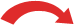# Convert Fractions to Percents

Divide the top of the fraction by the bottom, multiply by 100 and add a "%" sign.

## The Simplest Method is to Use a CalculatorSteps: Divide the top of the fraction by the bottom, Then multiply the result by 100 and read off the answer !

### Example: What is 5 8 as a percent?

Get your calculator and type in "5 ÷ 8 =", the calculator should show 0.625

(Remember to put the "%" sign so people know it is "per 100")

OR you can multiply the top by 100 first then divide by the bottom number:

### Example: What is 5 8 as a percent?

Multiply 5 by 100 first, then divide by 8

500 ÷ 8 = 62.5%

(Remember that "%" sign!)

## Or Move the Decimal 2 Places

After dividing, instead of multiplying by 100 we can just move the decimal point 2 places to the right, then add the %

### Example: Convert 1/8 to a percentage

Divide 1 by 8:

1 ÷ 8 = 0.125

Move the decimal point 2 places to the rightRemember to add the "%" sign: 12.5%

## Another (Harder) Method

Percent means "per 100", so try to change the fraction to  ? 100  form.

 Step 1: Find a number you can multiply the bottom of the fraction by to get 100. Step 2: Multiply both top and bottom of the fraction by that number. Step 3. Then write down just the top number with the "%" sign.

### Example 1: Convert 3 4 to a Percent

Step 1: We can multiply 4 by 25 to become 100

(why 25? because 100 divided by 4 is 25)

Step 2: Multiply top and bottom by 25:

 ×253 4 = 75 100×25

Step 3: Write down 75 with the percent sign:

### Example 2: Convert 3 16 to a Percent

Step 1: We have to multiply 16 by 6.25 to become 100

(why 6.25? because 100 divided by 16 is 6.25)

Step 2: Multiply top and bottom by 6.25:

 ×6.253 16 = 18.75 100×6.25

Step 3: Write down 18.75 with the percentage sign:

## Yet Another Method: Proportions

Because a percent is actually a ratio (parts per 100) we can also use Proportions to do the conversion.

First, put what you know into this form:

Top of Fraction Bottom of Fraction   =   Percent 100

Then solve using "multiply across the known corners, divide by the third number":

### Example: Convert 3 16 to percent

Fill in what you know:

3 16   =   Percent 100

Multiply across the known corners, then divide by the third number. The "known corners" are top left and bottom right:

Percent = (3 × 100) / 16
= 300 / 16
= 18.75%